### 闵科夫斯基距离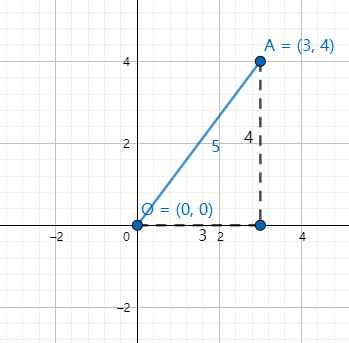$\sqrt{(x_1-y_1)^2+(x_2-y_2)^2}$

$d(x,y)=\sqrt{\sum_{i=0}^n(x_i-y_i)^2}$

$d_p(x,y)=(\sum_{i=0}^n|x_i-y_i|^p)^\frac{1}{p}$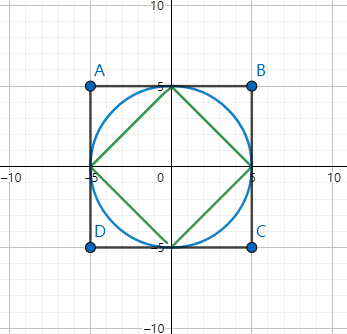### 余弦距离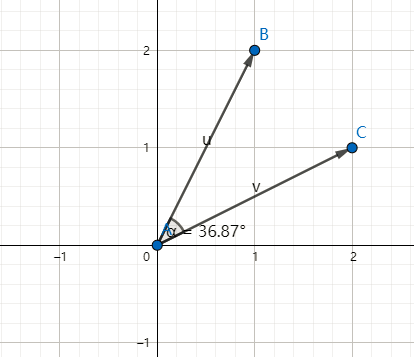$设x,y是两个向量，d(x,y)=\frac{x\bullet y}{||x||||y||}，即为向量的点积除以模的积。$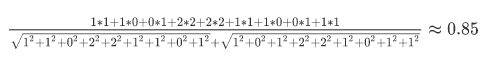### 马哈拉诺比斯距离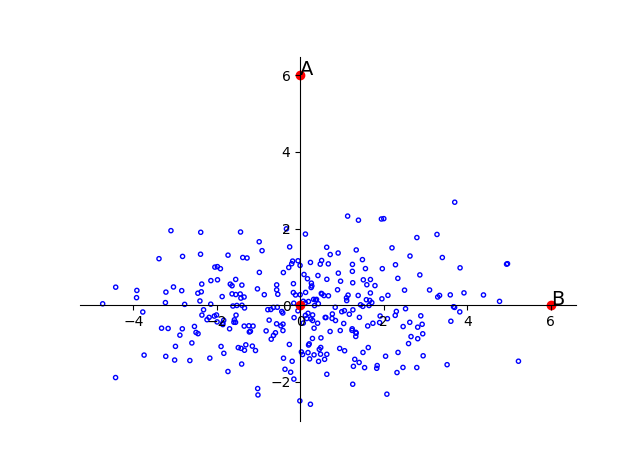$D_M(\vec{x})=\sqrt{(\vec{x}-\vec\mu)^TS^{-1}(\vec{x}-\vec\mu)}$

### 杰卡德距离

$J(X,Y)=\frac{|X \cap Y|}{|X \cup Y|} = \frac{2}{4} = 0.5$